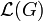# Nilpotent multiplier of abelian group is graded component of free Lie ring

## Statement

Suppose$G$ is an abelian group and$c$ is a positive integer. Denote by$\mathcal{L}(G)$ the free Lie ring on$G$. Note that$\mathcal{L}(G)$ naturally has the structure of a graded Lie ring. The claim is that the$c$-nilpotent multiplier$M^{(c)}(G)$ (defined as the Baer invariant of$G$ with respect to the subvariety of the variety of groups given by groups of nilpotency class at most$c$) is isomorphic, as an abelian group, to the$(c+1)^{th}$ graded component of$\mathcal{L}(G)$:$M^{(c)}(G) \cong (c+1)^{th} \mbox{ graded component of } \mathcal{L}(G)$

Note that the first graded component is$G$, so we get that:$\mbox{Additive group of } \mathcal{L}(G) \cong G \oplus \bigoplus_{c=1}^\infty M^{(c)}(G)$

### Interpretation in Schur functor terms

The graded components of the free Lie ring of$G$ can be viewed as functors of$G$. Each of these functors can explicitly described in Schur functor terms: see induction formula for Lie operad.

## Particular cases

Value of$c$ Term for group of class at most$c$ Term for$c$-nilpotent multiplier Statement How it follows
1 abelian group Schur multiplier$M(G)$ Schur multiplier of abelian group is its exterior square We have$M(G) = M^{(1)}(G)$ is the degree two graded component of$\mathcal{L}(G)$. This can be described as the quotient of$G \otimes G$ by all the degree two relations. The degree two relations are all generated by alternation, so the quotient is the exterior square$G \wedge G$.
2 group of nilpotency class two 2-nilpotent multiplier$M^{(2)}(G)$ Explicit description of 2-nilpotent multiplier of abelian group in terms of relations of the tensor cube$M^{(2)}(G)$ is the degree three graded component of$\mathcal{L}(G)$. This is a quotient of$G \otimes G \otimes G$ by a bunch of relations: alternation in the inner two variables and Jacobi identity in all three variables. The quotient by these relations is the 2-nilpotent multiplier.